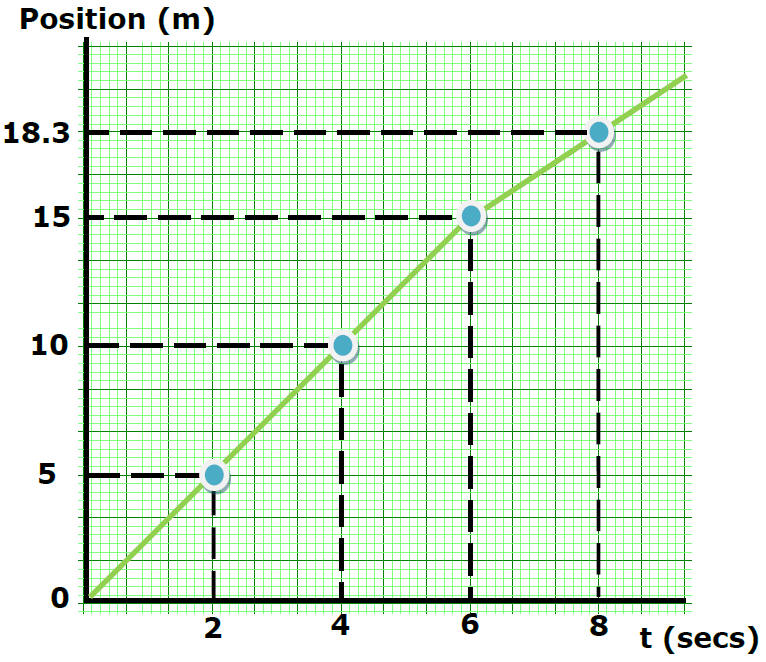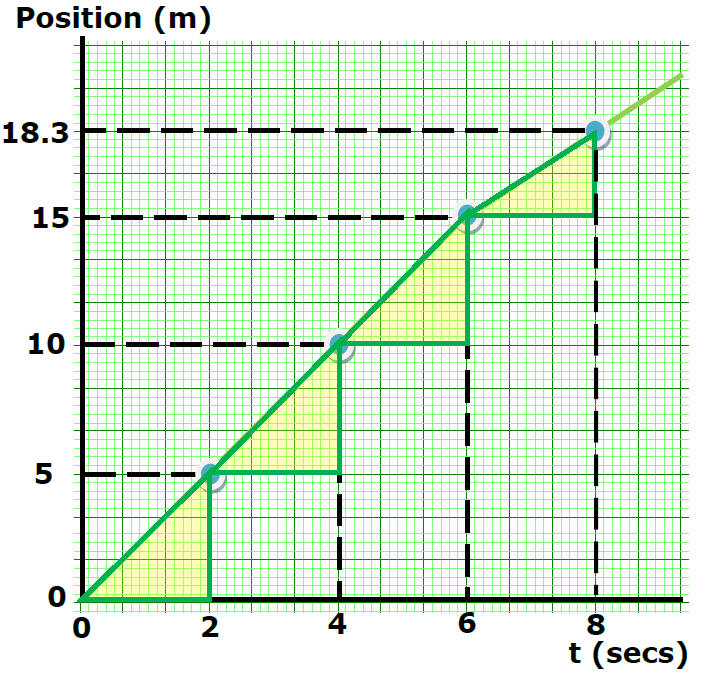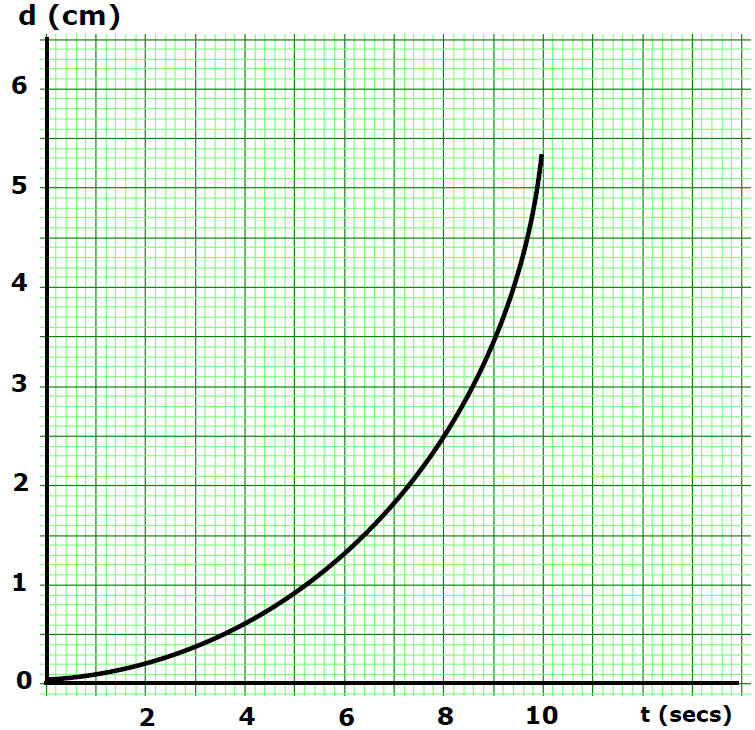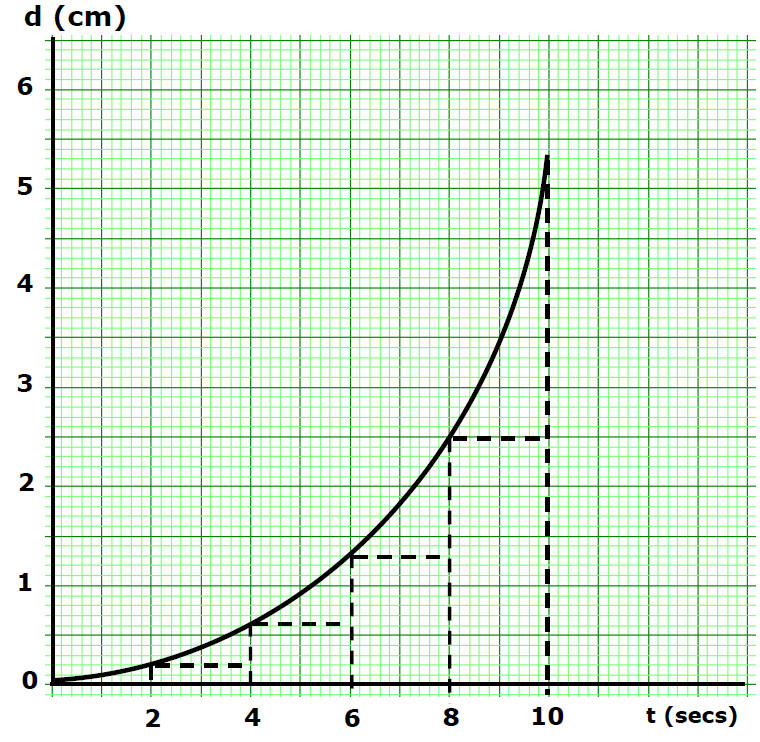HomeVideo Instruction

showmethephysics.com

 Motion Plots     When physicists want detailed information about the movement of an object, they gather data about the object each second or millisecond. Sometimes they gather the displacement (distance and direction from a starting point) at each moment in time and other times it is more useful to record the object's velocity.     The data collected is then plotted with the independent variable, time, on the x axis and either the object's distance and direction or its velocity on the vertical axis. Plotting this data gives the physicist a visual representation of the object's motion but the slope of a motion plot provides even more information.     Here's how it works. You'll probably remember that the slope of any plot is ΔY/ΔX. Therefore the slope of a displacement vs time plot at any given period of time would tell you about the velocity (Δd/Δt) of the object during the interval you are concerned with. On the other hand, the slope of a velocity vs time plot during a period time would reveal the object's acceleration. This is because acceleration is ΔV/Δt.

Fill in the Blank Notes

2nd way to describe motion

B) Motion Plots

(A. K. A.  Motion Diagrams)Position Vs Time Plot

Movement in a Straight linePosition (m) Time (sec) 0 2 4 6 8

 Position (m) Time (sec) 0 0 5 2 10 4 15 6 18.3 8Motion Map5m in 2 sec/5m in 2 sec/5m in 2 sec/3.3 m in 2 secSpeed from 0 to 2 seconds?Speed = Δd/Δt

= 5 m/2 sec

= 2.5 m/s

Speed from 2 to 4 seconds?Speed = Dd/Dt

= 5.0 m/2.0 sec

= 2.5 m/s

Speed from 4 to 6 seconds?Speed = Δd/Δt

= 5.0 m/2.0 sec

= 2.5 m/s

Does the object travel faster

or slower from 6 to 8 seconds?Slower Speed
(smaller change in d
during same time)Compare speed from 6 to 8 sec
with speed from 8 to 9 secSame Speed, Same SlopeWhat kind of motion
produced this plot?Increasing Speed

Motion Map• EquationsSpeedVelocity

(speed and direction)Direction